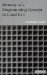# Exercises3.1 While compiling a C program, the GNUC compiler gave us the error message "warning: comparison is always true due to limited range of data type" . Does this message warrant a closer inspection of the statement it refers to, or can it be safely ignored? If you think that it cannot be safely ignored, give an example of where it may be essential.

3.2 Assume that the value of an int variable i is not zero. Is it possible that i+=3*i will set the value of i to zero? If so, under what circumstances?

3.3 Consider an int variable x and consider a pointer float* p = (float*)&x pointing to x . If we store a float value 2.35 in x directly using x = 2.35 or indirectly using *p = 2.35 , will we get the same bit pattern stored in x in both cases or instead different patterns?

3.4 Storing an int value in memory using a char* pointer can cause all kinds of errors. Can storing an int value in a char variable cause the same errors?

3.5 Calculate the size in bytes of the following structure:

` struct{      int b;      char a;      int c;    } `

3.6 In a C program, we store two short integers in an int variable; we then retrieve and display them later in the program:

` short* p;    int x;    ...    p = (short*) &x;    *p++ = 1;    *p=2;    ...    printf("first short=%d,second short=%d\n",*p, *(p+1)); `

During execution, the screen displays first short=1, second short=2 . What will the screen display if we compile and execute our program on a machine that has the opposite "endianess"?

3.7 Interpret the binary code 10010101010001010101010101000001 that is stored at the address 8023456. Assume that the machine is a "little endian" one; if you still have a problem with the interpretation, assume that the address is located in the static section of the address space rather than the dynamic data section.

3.8 In our C program we have

` char* p;    ...    p = "ab"; `

The compiler does not complain and the program works fine. If we change this to

` int* p;    ...    *p = 23; `

then will everything continue to work fine?

3.9 We are using a garbage collector with our C program. We know that, whenever a memory segment previously allocated is no longer referenced in our program, the garbage collector may deallocate it. In order to prevent an unwanted deallocation, we keep (in a special file) addresses of segments that we do not want to lose. Will this help us?

3.10 Since we did not want to make an error in counting of bytes, we used the code

` char *p;    ...    p = malloc(strlen("hello")+1);    strcpy(p,"hello"); `

` char *p;    ...    p = malloc(6);    strcpy(p,"hello"); `

Compare the memory requirements of each version: which one requires less memory?Memory as a Programming Concept in C and C++
ISBN: 0521520436
EAN: 2147483647
Year: 2003
Pages: 64

Similar book on Amazon